# 使用 Amazon SageMaker 运行基于 TensorFlow 的中文命名实体识别

## 二、中文命名实体识别算法

NER一直是自然语言处理（NLP）领域中的研究热点，从早期基于词典和规则的方法，到传统机器学习的方法，到近年来基于深度学习的方法，NER研究进展的大概趋势大致如下图所示。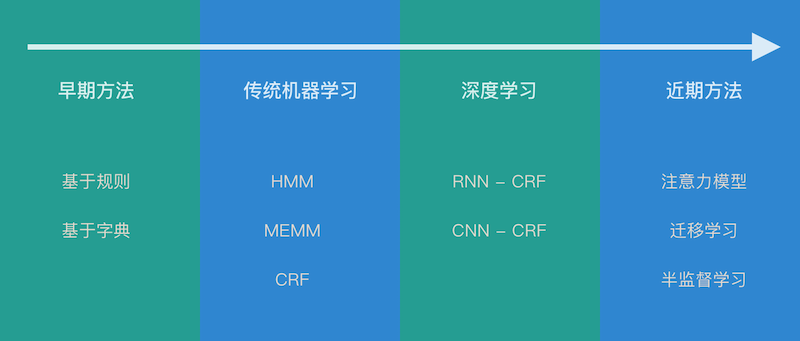BERT的全称是Bidirectional Encoder Representation from Transformers，即双向Transformer的编码器（Encoder），因为解码器（Decoder）是不能获得要预测的信息的。模型的主要创新点都在预训练方法上，即用了Masked LM和Next Sentence Prediction两种方法分别捕捉词语和句子级别的表示。

ALBERT（见参考资料4）基于BERT，但有一些改进，它可以在主要基准测试上获得最先进的性能，而参数却减少了30％。比如，对于albert_base_zh，它只有原始BERT模型的10%的参数，但是保留了主要精度。

## 三、在Amazon SageMaker中运行TensorFlow

### 1. 在Amazon SageMaker Notebook上运行TensorFlow开源代码

1.1 创建Amazon SageMaker Notebook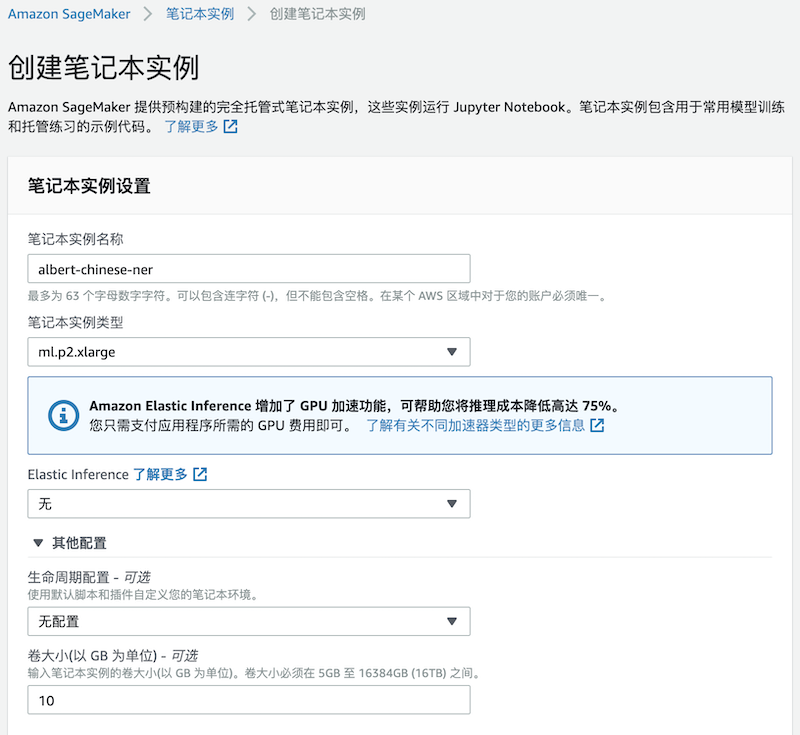1.2 下载代码和数据

``````cd ~/SageMaker
git clone https://github.com/whn09/albert-chinese-ner.git
``````

``````cd ~/SageMaker/albert-chinese-ner

1.3 运行代码

``````cd ~/SageMaker/albert-chinese-ner
source activate tensorflow_p36
./run_train.sh
``````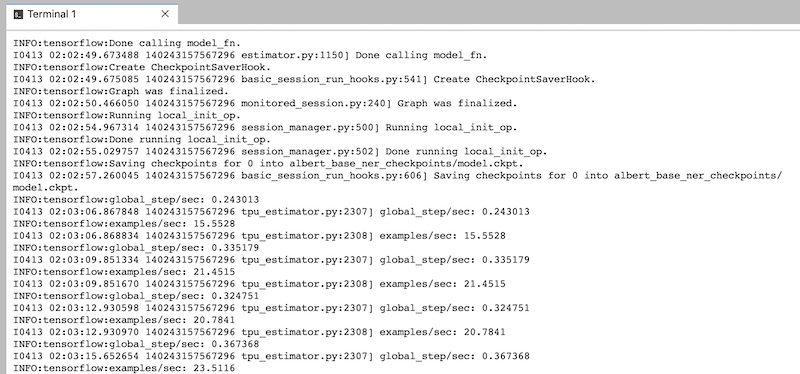### 2. 自定义容器（BYOC）

2.1 准备Dockerfile

``````FROM tensorflow/tensorflow:1.15.2-gpu-py3
ENV PATH="/opt/ml/code:\${PATH}"
COPY ./ /opt/ml/code
WORKDIR /opt/ml/code
``````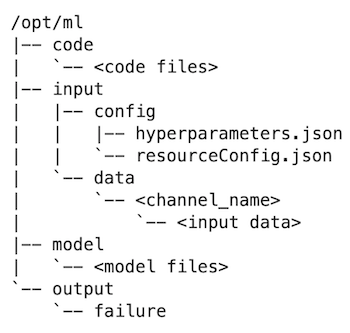2.2 创建训练的启动脚本

Amazon SageMaker在训练时默认的启动脚本是train，您可以将自己代码中的启动脚本命名为train，但我们更建议您使用我们提供的启动脚本train，该脚本是基于Python的，可以帮助您解析传入的超参数，并调用您代码中的实际启动脚本。

2.3 创建镜像并上传到Amazon ECR

2.4 本地测试

``````role = get_execution_role()

hyperparameters = {'task_name': 'ner', 'do_train': 'true', 'do_eval': 'true',  'data_dir': '/opt/ml/input/data/training', 'output_dir': '/opt/ml/model',                    'vocab_file': './albert_config/vocab.txt', 'bert_config_file': './albert_base_zh/albert_config_base.json', _seq_length': 128, 'train_batch_size': 64, 'learning_rate': 2e-5, 'num_train_epochs': 1}

instance_type = 'local'

if subprocess.call('nvidia-smi') == 0:
instance_type = 'local_gpu'

estimator = Estimator(role=role,
train_instance_count=1,
train_instance_type=instance_type,
image_name='sagemaker-tf-albert-chinese-ner:latest',
hyperparameters=hyperparameters)

estimator.fit('file:///home/ec2-user/SageMaker/albert-chinese-ner/data/')

``````

2.5 Amazon SageMaker测试

``````prefix = 'DEMO-tensorflow-albert-chinese-ner'
sess = sage.Session()
WORK_DIRECTORY = '/home/ec2-user/SageMaker/albert-chinese-ner/data'

client = boto3.client('sts')
account = client.get_caller_identity()['Account']
my_session = boto3.session.Session()
region = my_session.region_name
algorithm_name = 'sagemaker-tf-albert-chinese-ner'
ecr_image = '{}.dkr.ecr.{}.amazonaws.com/{}:latest'.format(account, region, algorithm_name)

hyperparameters = {'task_name': 'ner', 'do_train': 'true', 'do_eval': 'true', 'data_dir': '/opt/ml/input/data/training', 'output_dir': '/opt/ml/model', 'vocab_file': './albert_config/vocab.txt', 'bert_config_file': './albert_base_zh/albert_config_base.json', 'max_seq_length': 128, 'train_batch_size': 64, 'learning_rate': 2e-5, 'num_train_epochs': 3}

instance_type = 'ml.p2.xlarge'

estimator = Estimator(role=role,
train_instance_count=1,
train_instance_type=instance_type,
image_name=ecr_image,
hyperparameters=hyperparameters)

estimator.fit(data_location)
``````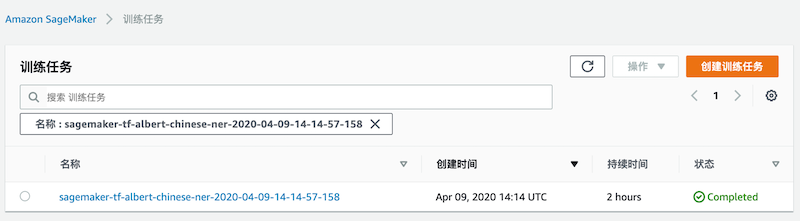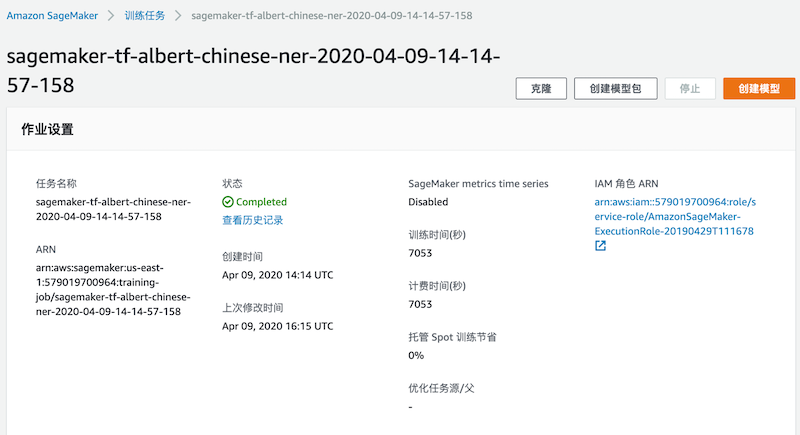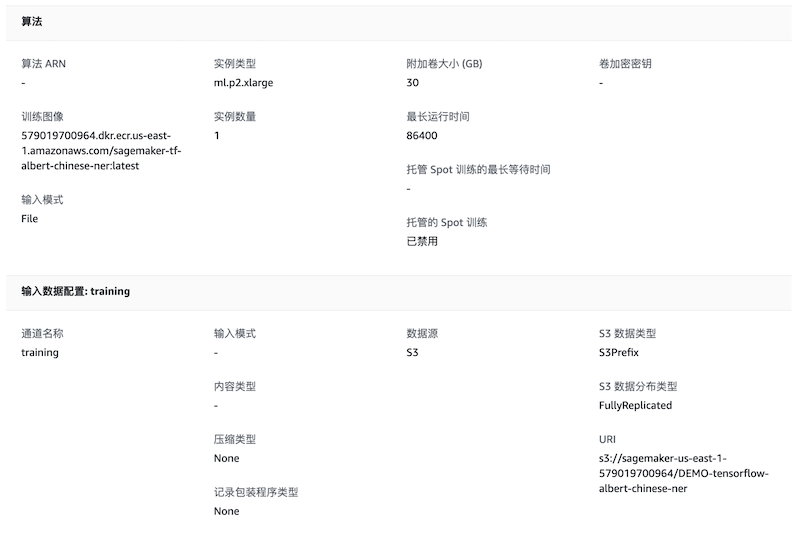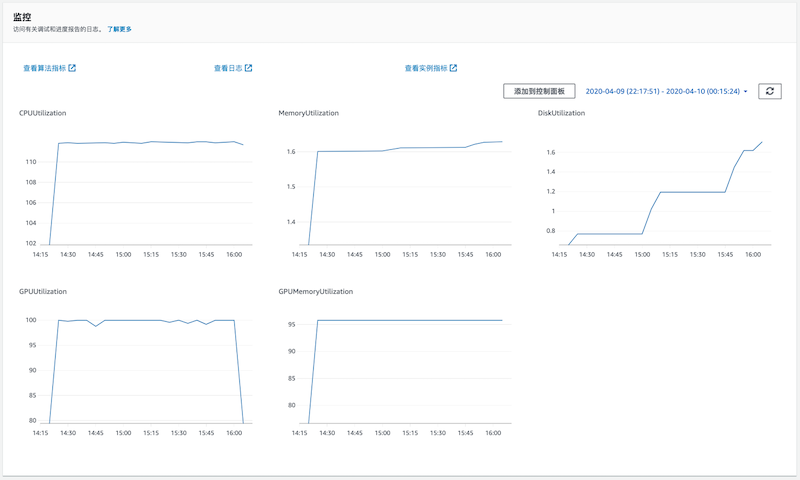2.6 训练结果

``````***** Eval results albert_base_ner_checkpoints/model.ckpt-0 *****
eval_f = 0.01672106
eval_precision = 0.010008455
eval_recall = 0.10698523
global_step = 0
loss = 2367.2935
***** Eval results albert_base_ner_checkpoints/model.ckpt-1000 *****
eval_f = 0.9256434
eval_precision = 0.95667034
eval_recall = 0.8976247
global_step = 1000
loss = 17.80814
***** Eval results albert_base_ner_checkpoints/model.ckpt-2000 *****
eval_f = 0.9444123
eval_precision = 0.94401
eval_recall = 0.9452899
global_step = 2000
loss = 10.842231
***** Eval results albert_base_ner_checkpoints/model.ckpt-2374 *****
eval_f = 0.948345
eval_precision = 0.9542561
eval_recall = 0.94261235
global_step = 2374
loss = 10.520088``````

### 3. 自定义脚本（BYOS）

3.1 本地测试

``````role = get_execution_role()

hyperparameters = {'task_name': 'ner', 'do_train': 'true', 'do_eval': 'true', _dir': '/opt/ml/input/data/training', 'output_dir': '/opt/ml/model', 'vocab_file': './albert_config/vocab.txt', 'bert_config_file': './albert_base_zh/albert_config_base.json', _seq_length': 128, 'train_batch_size': 64, 'learning_rate': 2e-5, 'num_train_epochs': 1}

train_instance_type='local'

if subprocess.call('nvidia-smi') == 0:
train_instance_type = 'local_gpu'

estimator = TensorFlow(entry_point='albert_ner.py',
source_dir='.',
train_instance_type=train_instance_type,
train_instance_count=1,
hyperparameters=hyperparameters,
role=role,
framework_version='1.15.2',
py_version='py3',
script_mode=True)

inputs = {'training': f'file:///home/ec2-user/SageMaker/albert-chinese-ner/data/'}

estimator.fit(inputs)
``````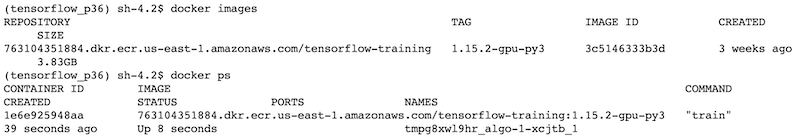3.2 Amazon SageMaker测试

``````inputs = sagemaker.Session().upload_data(path='/home/ec2-user/SageMaker/albert-chinese-ner/data', key_prefix='DEMO-tensorflow-albert-chinese-ner')

estimator = TensorFlow(entry_point='albert_ner.py',
source_dir='.',
train_instance_type='ml.p2.xlarge',
train_instance_count=1,
hyperparameters=hyperparameters,
role=role,
framework_version='1.15.2',
py_version='py3',
script_mode=True)

estimator.fit({'training': inputs})

``````

``````git_config = {'repo': 'https://github.com/whn09/albert-chinese-ner.git', 'branch': 'master'}

estimator = TensorFlow(entry_point='albert_ner.py',
source_dir='.',
git_config=git_config,
train_instance_type='ml.p2.xlarge',
train_instance_count=1,
hyperparameters=hyperparameters,
role=role,
framework_version='1.15.2',
py_version='py3',
script_mode=True)

estimator.fit({'training': inputs})
``````

## 参考资料

1. 本文代码：https://github.com/whn09/albert-chinese-ner
2. 使用预训练语言模型ALBERT做中文NER：https://github.com/ProHiryu/albert-chinese-ner
3. 海量中文预训练ALBERT模型：https://github.com/brightmart/albert_zh
4. ALBERT论文：https://arxiv.org/pdf/1909.11942.pdf
5. 命名实体识别 – Named-entity recognition | NER：https://easyai.tech/ai-definition/ner/

## 本篇作者